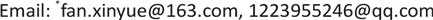﻿ 基于Navier-Stokes方程分析飞机抖振问题 A Class of Spectral Method for Solving Unsteady Navier-Stokes Equations

Pure Mathematics
Vol.08 No.04(2018), Article ID:26026,9 pages
10.12677/PM.2018.84060

A Class of Spectral Method for Solving Unsteady Navier-Stokes Equations

Jiansen Li, Jun Liu, Jiao Zhang, Xinyue Fan*

School of Mathematics and Statistics, Guizhou University, Guiyang GuizhouReceived: Jun. 30th, 2018; accepted: Jul. 15th, 2018; published: Jul. 23rd, 2018ABSTRACT

This paper focuses on the issue about using numerical computation to solve unsteady Navier-Stokes equations. We present a scheme called nonlinear Galerkin-Legendre Spectral method, which combines the Legendre spectral method with nonlinear Galerkin-Legendre method. Therefore, it is not necessary to meet Babuska-Brezzi inequality condition in the velocity space and pressure space. In this paper, we give a spectral scheme of nonlinear Galerkin-Legendre methods for solving 2D N-S equations. What’s more, we also provide the error estimation and proof the stability of this scheme. The method based on the separate calculation of viscous flow and potential flow, was used to solve the problem of plane buffeting, and has been replaced by the method based on the incompressible N-S equations. Therefore, it is necessary to use the spectral method to study the chattering problem of aircraft and to reduce the resistance by error analysis and to save energy.

Keywords:Navier-Stokes Equation, Nonlinear Galerkin Method, Buffet Problem, Legendre Spectral Method, Error Estimation1. 引言

2. 经典Galerkin方法下的结论

2.1. 预备知识

$a\left(u,v\right)={\int }_{\Omega }{u}_{x}{v}_{x}\text{d}x\text{}\left(u,v\in {H}_{0}^{1}\left(\Omega \right)\right)$

$a\left(u,v,w\right)=\frac{1}{2}{\int }_{\Omega }\sum _{i,j=1}^{n}\left[{u}_{i}\frac{\partial {v}_{j}}{\partial {x}_{i}}{w}_{j}-{u}_{i}\frac{\partial {w}_{j}}{\partial {x}_{i}}{v}_{j}\right]\text{d}x\text{\hspace{0.17em}}\text{\hspace{0.17em}}\text{\hspace{0.17em}}\text{\hspace{0.17em}}\left(u,v,w\in {H}_{0}^{1}\left(\Omega \right)\right)$

$a\left(u,v,w\right)=-a\left(u,v,w\right);\text{}a\left(u,v,v\right)=0$

$a\left(u,v,w\right)=C{‖u‖}_{{H}_{0}^{1}}{‖v‖}_{{H}_{0}^{1}}{‖w‖}_{{L}^{2}\left(\Omega \right)};\text{}\forall u,v\in {H}_{0}^{1}\left(\Omega \right),\text{}w\in {L}^{2}\left(\Omega \right)$

2.2. Galerkin方法的弱形式

$\Omega$ 是一个有界区域。考虑下面定常N-S方程  ：

$\left\{\begin{array}{l}{u}_{t}-\nu \Delta u+u\cdot \nabla u+\nabla p=f\text{\hspace{0.17em}}\text{\hspace{0.17em}}\text{\hspace{0.17em}}\left(在\Omega 中\right)\\ \nabla \cdot u=0\text{\hspace{0.17em}}\text{\hspace{0.17em}}\text{\hspace{0.17em}}\left(在\Omega 中\right)\\ u=0\text{\hspace{0.17em}}\text{\hspace{0.17em}}\text{\hspace{0.17em}}\left(在\partial \Omega 上\right)\end{array}$ (1)

$X={H}_{0}^{1}\left(\Omega \right),\text{\hspace{0.17em}}M={L}_{0}\left(\Omega \right)=\left\{q\in {L}^{2}\left(\Omega \right);{\int }_{\Omega }q\text{d}x=0\right\}$

$\left({u}_{t},v\right)+a\left(u,v\right)+{a}_{1}\left(u,u,v\right)-b\left(p,v\right)+b\left(q,u\right)=\left(f,v\right)$

$\forall \left(v,q\right)\in \left(X,M\right)$

$a\left(u,v\right)=\nu {\int }_{\Omega }\nabla u\cdot \nabla v\text{d}x,\text{}b\left(q,v\right)={\int }_{\Omega }qdivv\text{d}x$,

$\left(f,v\right)={\int }_{\Omega }f\cdot v\text{d}x$ .

${X}_{N}$${M}_{N}$ 分别是X和M空间中次数不超过N的多项式空间，则(1)的谱格式为：

$\left\{\begin{array}{l}\left({u}_{t},{v}_{N}\right)+a\left({u}_{N},{v}_{N}\right)+{a}_{1}\left({u}_{N},{u}_{N},{v}_{N}\right)-b\left({p}_{N},{v}_{N}\right)=\left(f,{v}_{N}\right)\\ \left(\nabla \cdot {u}_{N},{q}_{N}\right)=0,\text{}\forall {v}_{N}\in {X}_{N},\text{}{q}_{N}\in {M}_{N}\end{array}$ (2)

${‖u-{u}_{N}‖}_{1}+{\beta }_{N}{‖p-{p}_{N}‖}_{{L}^{2}}\le {N}^{1-m}{‖u‖}_{m}+{N}^{-s}{‖u‖}_{s}$

3. 非线性Galerkin-Legendre谱方法

3.1. 准备知识

${S}_{N}=span\left\{{L}_{0}\left(x\right),{L}_{1}\left(x\right),\cdots ,{L}_{N}\left(x\right)\right\}$ ,

${Q}_{2N}=span\left\{{L}_{N-1}\left(x\right),{L}_{N}\left(x\right),\cdots ,{L}_{2N}\left(x\right)\right\}$ ,

${W}_{2N}=\left\{w\in {Q}_{2N}/w\left(±1\right)=0\right\}$ ,

${S}_{2N}={Q}_{2N}\oplus {S}_{N-2}=span\left\{{L}_{0}\left(x\right),{L}_{1}\left(x\right),\cdots ,{L}_{2N}\left(x\right)\right\}$ ,

${V}_{2N}={V}_{N}\oplus {W}_{2N}=\left\{v\in {S}_{2N}/v\left(±1\right)=0\right\}$ .

${P}_{N}$${L}^{2}\left(\Omega \right)\to {V}_{N}$ 的正交投影算子，即

$\left(u-{P}_{N}u,v\right)=0$ , $\forall v\in {S}_{N}$ , $u\in {L}^{2}\left(\Omega \right)$

${\Pi }_{N}$${H}_{0}^{1}\left(\Omega \right)\to {V}_{N}$ 的投影算子，即

$a\left(u-{\Pi }_{N}u,v\right)=0$ , $\forall v\in {S}_{N}$ , $u\in {H}_{0}^{1}\left(\Omega \right)$

3.2. 非线性Galerkin-Legendre方法

$\left\{\begin{array}{l}\frac{\text{d}}{\text{d}t}\left({u}_{N},\varphi \right)+a\left({u}_{N},\varphi \right)+{a}_{1}\left({u}_{N}+{u}_{2N},{u}_{N}+{u}_{2N},\varphi \right)-b\left({p}_{N},\varphi \right)\\ =\left(f,\varphi \right)\text{,}\forall \varphi \in {V}_{N}\\ a\left({u}_{2N},\omega \right)+{a}_{1}\left({u}_{N}+{u}_{2N},{u}_{N},\omega \right)-b\left({p}_{2N},\omega \right)=\left(f,\omega \right)\\ \left(q,\nabla \cdot v\right)=0\text{,}\forall \omega \in {W}_{2N}\end{array}$ (3)

${‖{u}_{N}‖}^{2}\le C\left({‖u‖}_{1}^{2}+{‖f‖}^{2}\right)$

$‖u-{u}_{N}-{u}_{2N}‖\le C{N}^{1-s}+K\Delta {t}^{2}$ , $‖p-{p}_{N}-{p}_{2N}‖\le M{N}^{\frac{d-1}{2}-m}$

$\left\{\begin{array}{l}\frac{\text{d}}{\text{d}t}\left(u,\varphi +\omega \right)+\nu \left({\left({\prod }_{2N}u\right)}_{x},{\left(\varphi +\omega \right)}_{x}\right)+{a}_{1}\left(u,u,\varphi +\omega \right)\\ =\left(f,\varphi +\omega \right),\text{}\forall \varphi \in {V}_{N},\omega \in {W}_{2N}\\ b\left(q,\nabla \cdot \left(\varphi +\omega \right)\right)=0,\text{}\forall \varphi \in {V}_{N},\omega \in {W}_{2N}\end{array}$

$e={\prod }_{2N}u-\left({u}_{N}+{u}_{2N}\right)$ , $\xi ={\prod }_{N}u-{u}_{N}$ , $\eta ={\prod }_{2N}u-{u}_{N}-u{}_{2N}$ .

${‖e‖}_{1}^{2}={‖\xi ‖}_{1}^{2}+{‖\eta ‖}_{1}^{2}$

$\begin{array}{l}\frac{\text{d}}{\text{d}t}\left(u,\varphi +\omega \right)-\frac{\text{d}}{\text{d}t}\left({u}_{N},\varphi \right)+a\left(e,\varphi +\omega \right)+{a}_{1}\left(u,u,\varphi \right)\\ \text{ }-{a}_{1}\left({u}_{N}+{u}_{2N},{u}_{N}+{u}_{2N},\varphi \right)+{a}_{1}\left(u,u,\omega \right)-{a}_{1}\left({u}_{N}+{u}_{2N},{u}_{N},\omega \right)=0\end{array}$

$\begin{array}{l}a\left(e,\varphi +\omega \right)+\left({\xi }_{t},\varphi \right)\\ =\left[{a}_{1}\left({u}_{N}+{u}_{2N},{u}_{N}+{u}_{2N},\varphi \right)-{a}_{1}\left(u,u,\varphi \right)\right]\\ \text{\hspace{0.17em}}\text{\hspace{0.17em}}\text{ }+\left[{a}_{1}\left({u}_{N}+{u}_{2N},{u}_{N},\omega \right)-{a}_{1}\left(u,u,\omega \right)\right]+\left({\left({\Pi }_{N}u-u\right)}_{t},\varphi \right)-\left({u}_{t},\omega \right)\end{array}$

$\begin{array}{c}{‖e‖}_{1}^{2}+\frac{1}{2}\frac{\text{d}}{\text{d}t}{‖\xi ‖}^{2}=\left[{a}_{1}\left({u}_{N}+{u}_{2N},{u}_{N}+{u}_{2N},\xi \right)-{a}_{1}\left(u,u,\xi \right)\right]\\ \text{\hspace{0.17em}}\text{\hspace{0.17em}}+\left[{a}_{1}\left({u}_{N}+{u}_{2N},{u}_{N}+{u}_{2N},\eta \right)-{a}_{1}\left(u,u,\eta \right)\right]\\ \text{\hspace{0.17em}}\text{\hspace{0.17em}}+\left[\left({\left({\Pi }_{N}u-u\right)}_{t},\xi \right)-\left({u}_{t},\eta \right)\right]\\ \triangleq {I}_{1}+{I}_{2}+{I}_{3}\end{array}$

$\begin{array}{c}{I}_{1}={a}_{1}\left({u}_{N}+{u}_{2N},{u}_{N}+{u}_{2N},\xi \right)-{a}_{1}\left(u,u,\xi \right)\\ =-{a}_{1}\left({u}_{N}+{u}_{2N},u-{\prod }_{2N}u,\xi \right)-{a}_{1}\left({u}_{N}+{u}_{2N},e,\xi \right)-{a}_{1}\left(u-{\prod }_{2N}u+e,u,\xi \right)\\ \triangleq {I}_{11}+{I}_{12}+{I}_{13}\end{array}$

$\begin{array}{c}{I}_{2}={a}_{1}\left({u}_{N}+{u}_{2N},{u}_{N},\eta \right)-{a}_{1}\left(u,u,\eta \right)\\ =-{a}_{1}\left({u}_{N}+{u}_{2N},u-{\prod }_{N}u,\eta \right)-{a}_{1}\left({u}_{N}+{u}_{2N},\xi ,\eta \right)-{a}_{1}\left(u-{\prod }_{2N}u+e,u,\eta \right)\\ \triangleq {I}_{21}+{I}_{22}+{I}_{23}\end{array}$

$\begin{array}{c}{I}_{13}+{I}_{23}=-{a}_{1}\left(u-{\prod }_{2N}u+e,u,e\right)\\ \le C\left({‖u-{\prod }_{2N}u‖}_{1}+{‖e‖}_{1}\right){‖u‖}_{1}‖e‖\\ \le \frac{\nu }{8}{‖e‖}_{1}^{2}+M\left({‖u-{\prod }_{2N}u‖}_{1}^{2}+{‖e‖}^{2}\right)\end{array}$

$\begin{array}{c}{I}_{11}+{I}_{21}={a}_{1}\left(e-{\prod }_{2N}u,u-{\prod }_{2N}u,e\right)+{a}_{1}\left(e-{\prod }_{N}u,{\prod }_{2N}u-{\prod }_{N}u,\eta \right)\\ \le \frac{\nu }{4}{‖e‖}_{1}^{2}+M\left({‖u-{\prod }_{2N}u‖}_{1}^{2}+{‖e‖}^{2}\right)+M{N}^{-2}{‖{\prod }_{N}u-{\prod }_{2N}u‖}_{1}^{2}\end{array}$

$\begin{array}{c}{I}_{3}=\left[\left({\left({\Pi }_{N}u-u\right)}_{t},\xi \right)-\left(\left(I-{P}_{N-2}\right){u}_{t},\eta \right)\right]\\ \le \frac{\nu }{8}\left({‖\xi ‖}_{1}^{2}+{‖\eta ‖}_{1}^{2}\right)+C\left({‖\left({\Pi }_{N}u-u\right)‖}_{-1}^{2}+{‖\left(I-{P}_{N-2}\right){u}_{t}‖}_{-1}^{2}\right)\\ \le \frac{\nu }{8}{‖e‖}_{1}^{2}+C\left({‖\left({\Pi }_{N}u-u\right)‖}_{-1}^{2}+{‖\left(I-{P}_{N-2}\right){u}_{t}‖}_{-1}^{2}\right)\\ \le \frac{\nu }{8}{‖e‖}_{1}^{2}+\Delta {t}^{2}{‖{u}_{t}‖}_{-1}^{2}\end{array}$

${‖e‖}_{1}^{2}+\frac{1}{2}\frac{\text{d}}{\text{d}t}{‖\xi ‖}^{2}\le M{‖e‖}^{2}+M{N}^{-2}{‖{\prod }_{N}u-{\prod }_{2N}u‖}_{1}^{2}+\Delta {t}^{2}{‖{u}_{t}‖}_{-1}^{2}$ .

$‖u-{u}_{N}-{u}_{2N}‖\le {N}^{1-s}{‖u‖}_{s}+\Delta {t}^{2}{‖{u}_{t}‖}_{-1}^{2}$

${V}_{N}=\left\{{v}_{N}\in {H}_{0}^{1}\left(\Omega \right)/\left({q}_{N},\nabla \cdot {v}_{N}\right)=0,\forall {q}_{N}\in {M}_{N}\right\}$ 在文献  中有以下结果：

${\beta }_{N}‖p-{p}_{N}-{p}_{2N}‖\le \underset{\begin{array}{l}\text{\hspace{0.17em}}\text{\hspace{0.17em}}{u}_{N}\in {V}_{N}\\ {u}_{2N}\in {W}_{2N}\end{array}}{\mathrm{inf}}|‖u-{u}_{N}-{u}_{2N}‖|+\underset{{q}_{N}\in {M}_{N}}{\mathrm{inf}}‖p-{q}_{N}‖$

(1)式减去(3)式

${‖e‖}_{1}^{2}-\left(p-{p}_{N}-{p}_{2N},\nabla \cdot {v}_{N}\right)=0$

$\begin{array}{l}{\beta }_{N}‖q-{p}_{N}-{p}_{2N}‖\le \underset{{v}_{N}\in {H}_{0}^{1}\left(\Omega \right)}{\mathrm{sup}}\frac{\left({q}_{N}-{p}_{N}-{p}_{2N},\nabla \cdot {v}_{N}\right)}{|‖{v}_{N}‖|}\\ =\underset{{v}_{N}\in {H}_{0}^{1}\left(\Omega \right)}{\mathrm{sup}}\frac{\alpha \left(u-{u}_{N}-{u}_{2N},{v}_{N}\right)+\nu \left(\nabla \left(u-{u}_{N}-{u}_{2N}\right),\nabla {v}_{N}\right)-\left(p-{q}_{N},\nabla \cdot {v}_{N}\right)}{|‖{v}_{N}‖|}\end{array}$

$\begin{array}{c}{\beta }_{N}‖q-{p}_{N}-{p}_{2N}‖\le {\beta }_{N}\mathrm{inf}\left\{‖p-{q}_{N}‖+‖{q}_{N}-{p}_{N}-{p}_{2N}‖\right\}\\ \le \underset{{q}_{N}\in {M}_{N}}{\mathrm{inf}}‖p-{q}_{N}‖+‖u-{u}_{N}-{u}_{2N}‖\end{array}$

${\beta }_{N}\ge C\left(\alpha ,\nu \right){N}^{-\frac{d-1}{2}}$ , $\left(d=2或3\right)$

${N}^{-\frac{d-1}{2}}‖p-{p}_{N}-{p}_{2N}‖\le {N}^{-m}{‖p‖}_{m}$

${|u-{\stackrel{˜}{u}}_{N}|}_{1}+{‖p-{\stackrel{˜}{p}}_{N}‖}_{{L}^{2}}\le C{N}^{1-s}+M{N}^{\frac{d-1}{2}-m}+K\Delta {t}^{2}$

4. 数值算例

$\left\{\begin{array}{l}{u}_{t}-\nu \Delta u+u\cdot \nabla u+\nabla p=0\text{}\left(在\Omega 中\right)\\ div\text{}u=0\text{}\left(在\Omega 中\right)\\ u=0\text{}\left(在\partial \Omega 上\right)\end{array}$ (4)

$\left(\frac{{u}_{N}^{k+1}-{u}_{N}^{k}}{\Delta t},{v}_{N}\right)+\nu \left({\left({u}_{N}^{k+1}\right)}_{x},{\left({v}_{N}\right)}_{x}\right)+\left({u}_{N}^{k+1}{\left({u}_{N}^{k}\right)}_{x},{v}_{N}\right)+\left(\frac{{p}_{N}^{k+1}-{p}_{N}^{k}}{\Delta t},{v}_{N}\right)=0$ (5)

${u}_{N}^{k}\approx \sum _{k=0}^{N-2}{\stackrel{˜}{u}}_{k}{\varphi }_{k}\left(x\right)$ ,

${p}_{N}^{k}\approx \sum _{k=0}^{N}{\stackrel{˜}{p}}_{k}{\varphi }_{k}$

${\stackrel{˜}{u}}_{k}$${\stackrel{˜}{p}}_{k}$ 是插值系数， ${\varphi }_{k}$ 是插值基函数。

$\left[\begin{array}{cc}\frac{1}{\Delta t}M+K& {D}^{\text{T}}\\ D& 0\end{array}\right]\left[\begin{array}{c}{U}^{k+1}\\ {p}^{k+1}-{p}^{k}\end{array}\right]=\left[\begin{array}{c}\frac{1}{\Delta t}M{U}^{k}-{D}^{\text{T}}{p}^{k}\\ 0\end{array}\right]$

$\left\{\begin{array}{l}\text{Step}\text{\hspace{0.17em}}\text{1}:\left(\frac{1}{\Delta t}M+K\right){\stackrel{˜}{U}}^{k+1}=\frac{1}{\Delta t}M{U}^{k}-{D}^{\text{T}}{p}^{k}\\ \text{Step}\text{\hspace{0.17em}}\text{2}:D{M}^{-1}{D}^{\text{T}}{\Phi }^{k+1}=\frac{1}{\Delta t}D{\stackrel{˜}{U}}^{k+1}\\ \text{Step}\text{\hspace{0.17em}}\text{3}:{U}^{k+1}={\stackrel{˜}{U}}^{k+1}-\Delta t{M}^{-1}{D}^{\text{T}}{\Phi }^{k+1}\\ \text{Step}\text{\hspace{0.17em}}\text{4}:{p}^{k+1}={\Phi }^{k+1}+{p}^{k}\end{array}$

$u\left(x,y,t\right)=\text{π}\mathrm{sin}t\left(\mathrm{sin}2\text{π}y{\mathrm{sin}}^{2}\text{π}x,-\mathrm{sin}2\text{π}x{\mathrm{sin}}^{2}\text{π}y\right)$

$p\left(x,y,t\right)=\mathrm{sin}t\mathrm{cos}\text{π}x\mathrm{sin}\text{π}y$Table 1. The comparison of error estimates for velocity in two different formats with N = 16 and T = 1Table 2. The comparison of error estimates for pressure in two different formats with N = 16 and T = 1Table 3. The error estimates and convergence order for different time intervals and N with T = 1 and T = 2

5. 结语

A Class of Spectral Method for Solving Unsteady Navier-Stokes Equations[J]. 理论数学, 2018, 08(04): 450-458. https://doi.org/10.12677/PM.2018.84060

1. 1. Temam, R. (2013) Navier-Stokes Equations, Theory and Numerical Analysis. AMS Chelsea Edition, American Mathematical Society, Rhode Island.

2. 2. Marion, M. and Temam, R. (1989) Nonlinear Galerkin Methods. SIAM Journal on Numerical Analysis, 2, 1139-1157. https://doi.org/10.1137/0726063

3. 3. 罗振东, 朱江, 王会军, 等. 定常的Navier-Stokes方程的非线性Galerkin/Petrov最小二乘混元法[J]. 应用数学和力学, 2011, 7(7): 697-887.

4. 4. Shen, J. and Wang, L.-L. (2011) Spectral Methods, Springer, Heideberg, Dordrecht, London, New York.

5. 5. 何银年, 黄艾香. 非定常N-S方程的非线性Galerkin算法及其误差估计[J]. 系统科学与数学, 1991, 19(1): 116-122.

6. 6. 向新民. 谱方法的数值分析[M]. 北京: 科学出版社, 2000: 153-156.

7. 7. France, L.P. and Hughes, T.J. (1998) Two Classes of Mixed Finite Element Methods. Computer Methods in Applied Mechanics and Engineering, 69, 89-129. https://doi.org/10.1016/0045-7825(88)90168-5

8. 8. 牟让科, 杨永年. 飞机抖振问题研究进展[J]. 应用力学学报, 2001, 18(s1): 143-149.

9. 9. Shen, J. and Yu, H. (2010) Efficient Spectral Sparse Grid Methods and Applications to High Dimensional Elliptic Problems. SIAM Journal on Scientific Computing, 32, 3228-3250. https://doi.org/10.1137/100787842

10. 10. 胡园园, 谢江, 张武, 等. 二维不可压缩Navier-Stokes方程的并行谱有限元法求解[J]. 计算机应用, 2017(1): 42-47

11. 11. Liu, J. and Fan, X.Y. (2017) Legendre-Galerkin Methods for Nonlinear Partial Differential Equations. Advance in Intelligent Systems Research, 23, 1951-6851. https://doi.org/10.2991/icmia-17.2017.71

12. NOTES

*通讯作者。